Question

# 5 Let f(x, y) What is the shortest distance d between a point on the surface...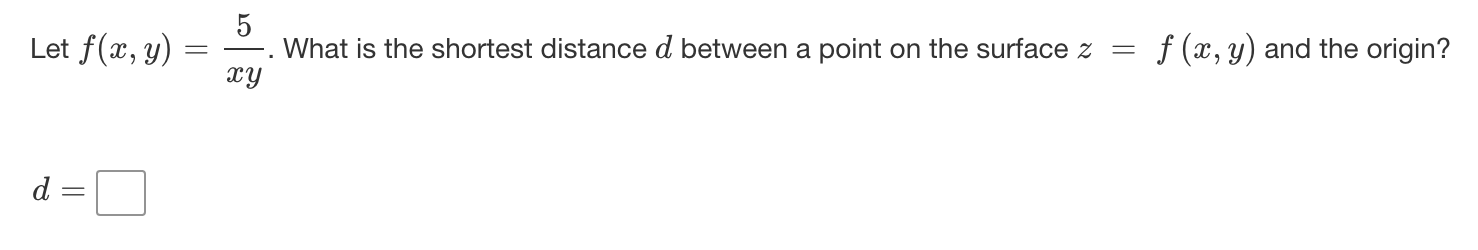5 Let f(x, y) What is the shortest distance d between a point on the surface z = f (x, y) and the origin? xy d =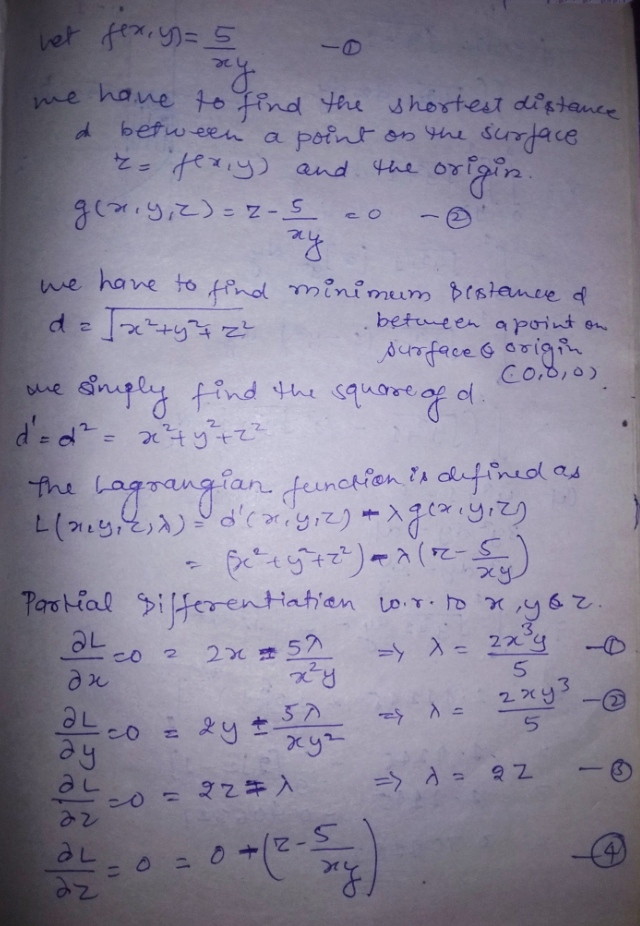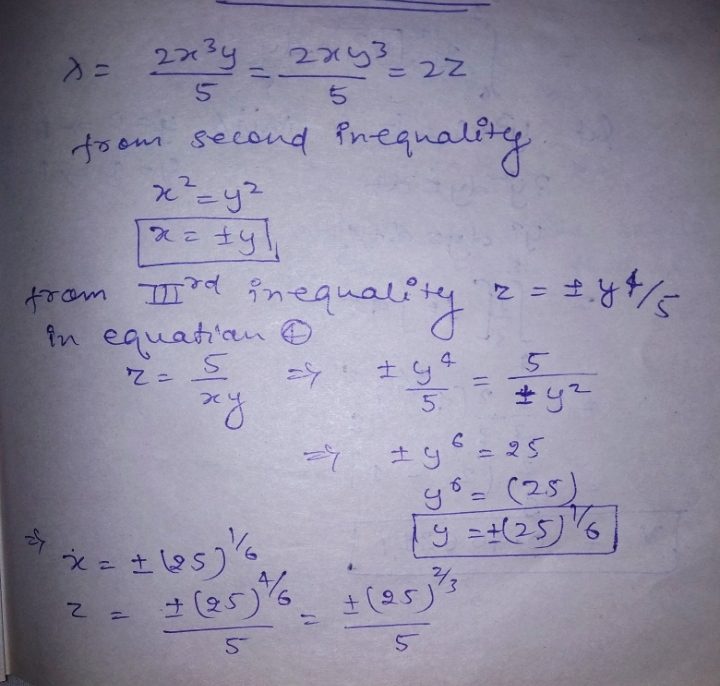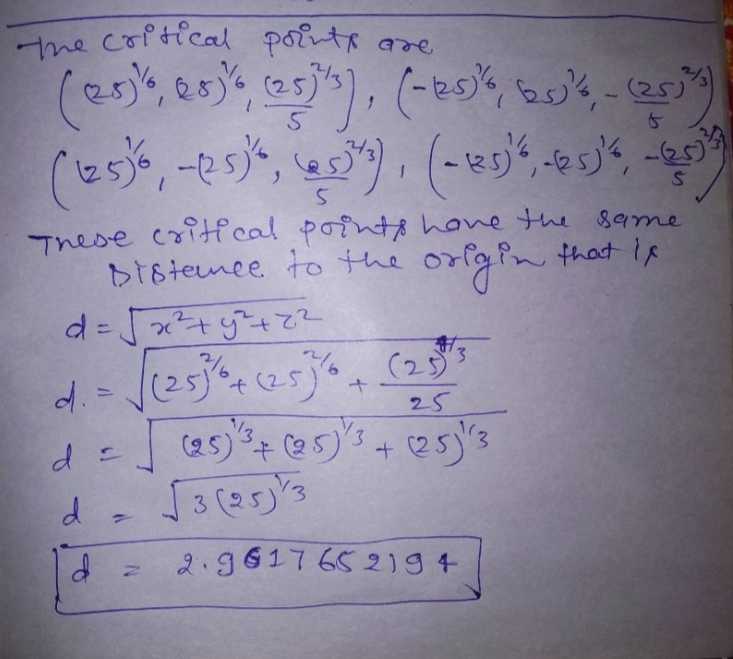#### Earn Coins

Coins can be redeemed for fabulous gifts.

Similar Homework Help Questions
• ### 3. Find the shortest distance from the center of the quadratic surface 9 x2+54 x +4...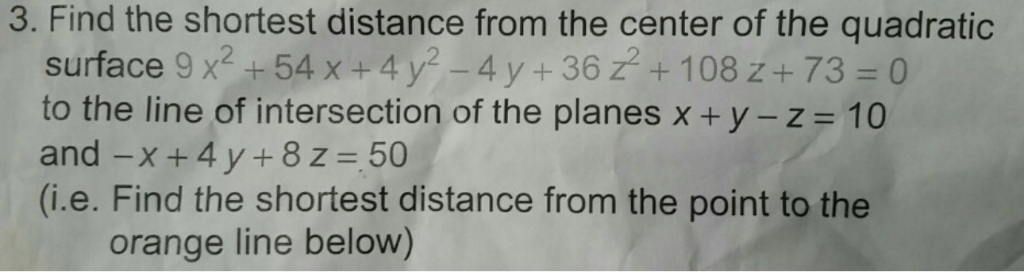3. Find the shortest distance from the center of the quadratic surface 9 x2+54 x +4 y-4 y + 36 z+ 108 z + 73 = 0 to the line of intersection of the planes x + y-z = 10 and -x + 4 y + 8 z = 50 (i.e. Find the shortest distance from the point to the orange line below) 3. Find the shortest distance from the center of the quadratic surface 9 x2+54 x +4 y-4...

• ### let f(x,y)=sqrt(49-x^2-y^2) (A) describe the cross sections of the surface Z=f(x,y) produced by cutting it with...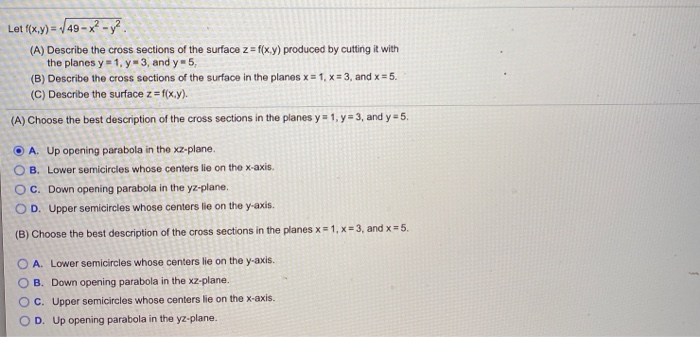let f(x,y)=sqrt(49-x^2-y^2) (A) describe the cross sections of the surface Z=f(x,y) produced by cutting it with the planes y=1, y=3, and y=5. (B) describe the cross sections of the surface in the planes x=1, x=3, and x=5. (C) describe the surface z=f(x,y). Let f(x,y) = 49 - x? -y?. (A) Describe the cross sections of the surface z=f(xy) produced by cutting it with the planes y = 1, y = 3, and y-5, (B) Describe the cross sections of the...

• ### x2 + y2. Find the shortest The distance between a point (cy) and the origin is...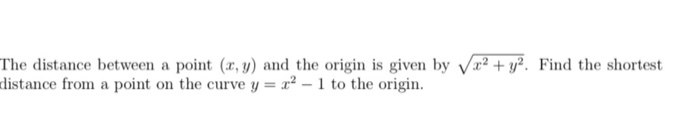x2 + y2. Find the shortest The distance between a point (cy) and the origin is given by distance from a point on the curve y = 2-1 to the origin.

• ### Y 14°42' To find the distance between a point X and an inaccessible point Z, a...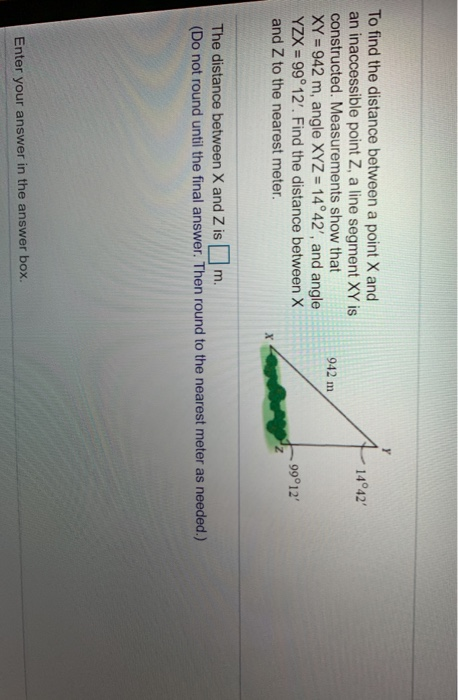Y 14°42' To find the distance between a point X and an inaccessible point Z, a line segment XY is constructed. Measurements show that XY = 942 m, angle XYZ = 14°42', and angle YZX = 99°12'. Find the distance between X and Z to the nearest meter. 942 m 99°12' X The distance between X and Z is m. (Do not round until the final answer. Then round to the nearest meter as needed.) Enter your answer in the...

• ### rty. I 5. [16 pointsj Consider the function f(x, y,z) Let S denote the level surface consisting of all points in space such that f(,y,z)-4, and let P- (2,-2,1), which is on S. a) Calculate Vf. b) Det...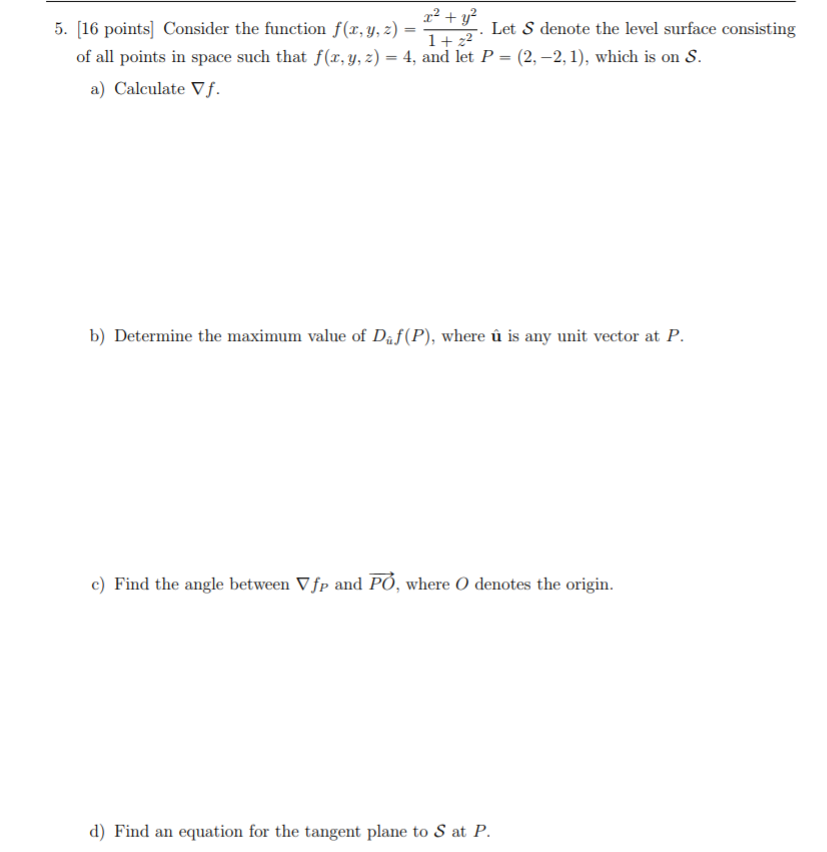rty. I 5. [16 pointsj Consider the function f(x, y,z) Let S denote the level surface consisting of all points in space such that f(,y,z)-4, and let P- (2,-2,1), which is on S. a) Calculate Vf. b) Determine the maximum value of Daf(P), where u is any unit vector at P c) Find the angle between Vfp and PO, where O denotes the origin. d) Find an equation for the tangent plane to S at P rty. I 5. [16...

• ### 3. (4 pts) Find the shortest distance from the surface xy + 3x + z2 =...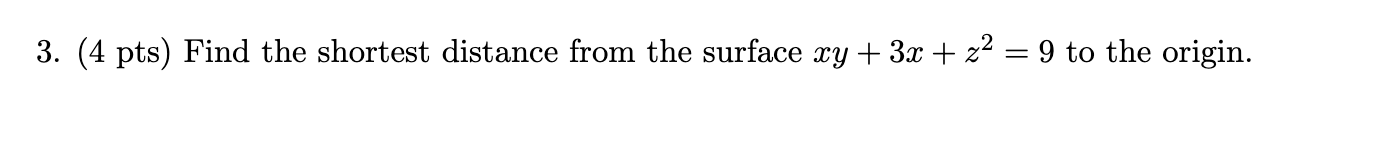3. (4 pts) Find the shortest distance from the surface xy + 3x + z2 = 9 to the origin.

• ### 3. (4 pts) Find the shortest distance from the surface xy + 3x + z2 =...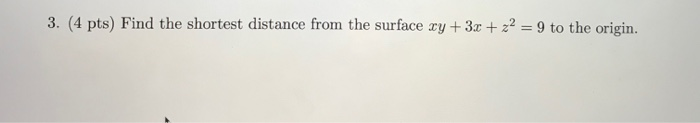3. (4 pts) Find the shortest distance from the surface xy + 3x + z2 = 9 to the origin.

• ### Let f(x, y) = x²y + 5yº. At the point (1, -2), which one is incorrect...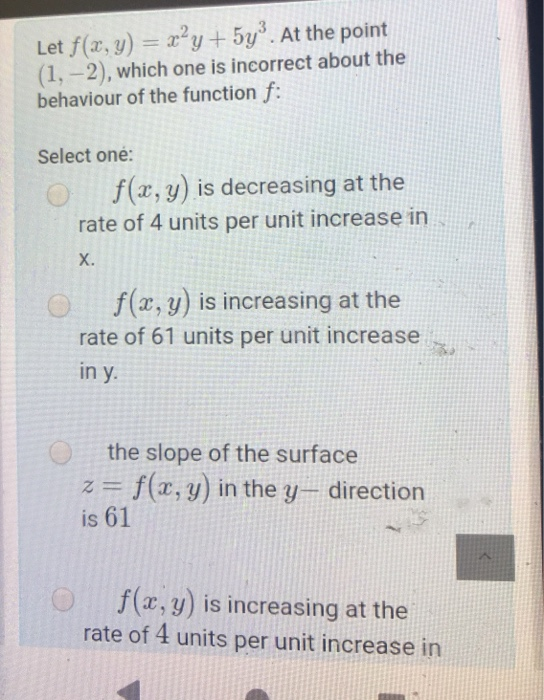Let f(x, y) = x²y + 5yº. At the point (1, -2), which one is incorrect about the behaviour of the function f: Select one: f(x, y) is decreasing at the rate of 4 units per unit increase in X. f(x, y) is increasing at the rate of 61 units per unit increase in y o the slope of the surface Z = f(x,y) in the y, direction is 61 f(x,y) is increasing at the rate of 4 units per...

• ### Find the area of the lateral surface over the curve C in 6. the xy-plane and under the surface z - f(x,y) f(x,y)-h, C:y-1 -x2 from (1,0) to (0,1) Surface: Lateral surface area - f(x, y) ds z =f(x, y)...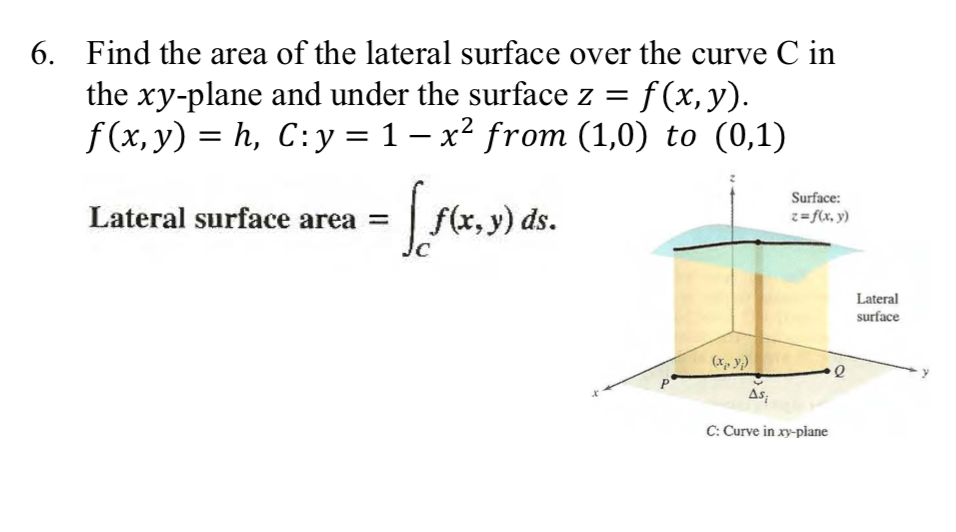Find the area of the lateral surface over the curve C in 6. the xy-plane and under the surface z - f(x,y) f(x,y)-h, C:y-1 -x2 from (1,0) to (0,1) Surface: Lateral surface area - f(x, y) ds z =f(x, y) Lateral surface xy) As C: Curve in xy-plane Find the area of the lateral surface over the curve C in 6. the xy-plane and under the surface z - f(x,y) f(x,y)-h, C:y-1 -x2 from (1,0) to (0,1) Surface: Lateral surface...

• ### 1. Let F(x, y, z) = (-y + ,2-2,2-y), and let S be the surface of...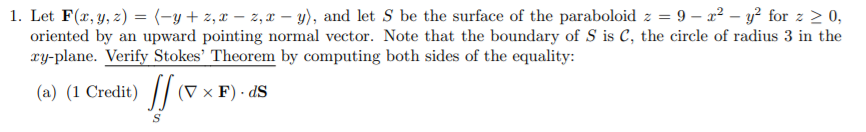1. Let F(x, y, z) = (-y + ,2-2,2-y), and let S be the surface of the paraboloid 2 = 9-32 - v2 for 2 > 0. oriented by an upward pointing normal vector. Note that the boundary of S is C, the circle of radius 3 in the xy-plane. Verify Stokes' Theorem by computing both sides of the equality: (a) (1 Credit) || (D x F). ds (b) (1 Credit) \$F. dr